Mathematics
Easy

Question

#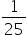= ______%

## 2550104Hint:

## The correct answer is: 4

### Convert fraction into decimal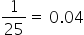,then multiply the number by 100 and add the symbol %.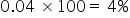Thus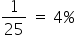Alternatively ,
Multiply the fraction by 100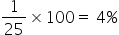### Related Questions to study#### With Turito Foundation.#### Get an Expert Advice From Turito.